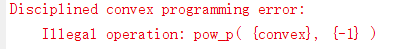# How to use normalized infinite norm to make the solution tend to 0 or 1

I need to solve a fitting problem and solve X according to the conditions Y =AX. Y is a M1 complex vector ,A is a MN complex Dictionary Matrix，X is a N1 complex vector. I want normalized X tend to be 0 or 1 by minimize ||x/max(x)-0.5||∞

cvx_begin
minimize( square_pos(norm(Y-AX),2)+ lambdanorm((X*inv_pos(max(abs(X)))-0.5),inf))
cvx_end

matlab gives messages error usingI think it is because the object inside inv_pos need to be concave,but max(X) is convex. Then how can i rewrite the normalized X？